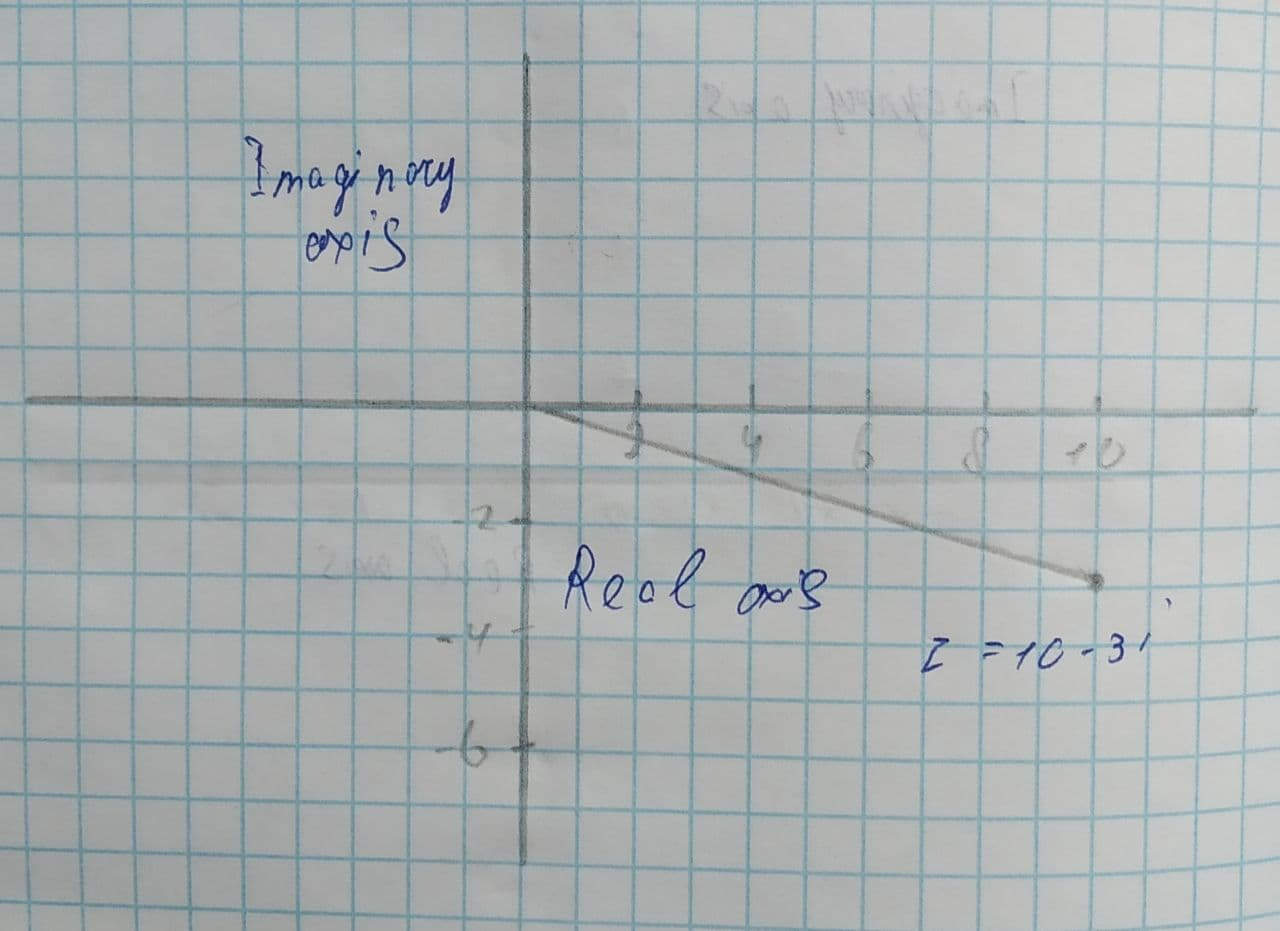# Plot the complex number z=10-3i and find its absolute value.Bergen 2021-07-28 Answered
Plot the complex number and find its absolute value.
z=10-3i

• Questions are typically answered in as fast as 30 minutes

### Plainmath recommends

• Get a detailed answer even on the hardest topics.
• Ask an expert for a step-by-step guidance to learn to do it yourself.Nicole Conner

Concept used:
The absolute value or modulus of complex number is the distance
between the origin (0,0) and point (a,b) in the complex plane.
Formula to find the absolute value of any complex number:
$$\displaystyle{\left|{z}\right|}=\sqrt{{{x}^{{{2}}}+{y}^{{{2}}}}}\ {\quad\text{or}\quad}\ {\left|{z}\right|}=\sqrt{{{a}^{{{2}}}+{b}^{{{2}}}}}$$.
To plot the graph:
Step 1. Determine the real and imaginary party of the complex number.
Step2. Move along the horizontal axis to show the real part of the number.
Step3. Move parallel to the vertical to show the imaginary part of the number.
Step4. Plot the point.
Calculation:
The complex number is Interprets as z=a+bi can be represent as the point (a,b) in the complex plane.
The complex number z=10-3i can be represented as the point (10, -3) in the complex plane.
The plot complex number z=10-3i as shown in below:The absolute value of z = a+bi is $$\displaystyle{\left|{a}+{b}{i}\right|}=\sqrt{{{a}^{{{2}}}+{b}^{{{2}}}}}$$.
$$\displaystyle{\left|{z}\right|}=\sqrt{{{10}^{{{2}}}+{\left(-{3}\right)}^{{{2}}}}}$$
$$\displaystyle\Rightarrow\sqrt{{{100}+{9}}}=\sqrt{{{109}}}$$.